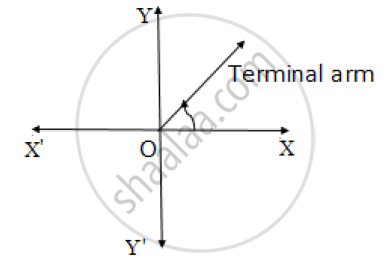# For the Angle in Standard Position If the Initial Arm Rotates 25° In Anticlockwise Direction, Then State the Quadrant in Which Terminal Arm Lies (Draw the Figure and Write the Answer). - Geometry

For the angle in standard position if the initial arm rotates 25° in anticlockwise direction, then state the quadrant in which terminal arm lies (Draw the figure and write the answer).

#### Solution

The initial arm rotates 25° in the anticlockwise direction.
The angle is positive and the measure of the angle is between 0° and 90°.
Thus, the terminal arm lies in quadrant I.Concept: Angles in Standard Position
Is there an error in this question or solution?